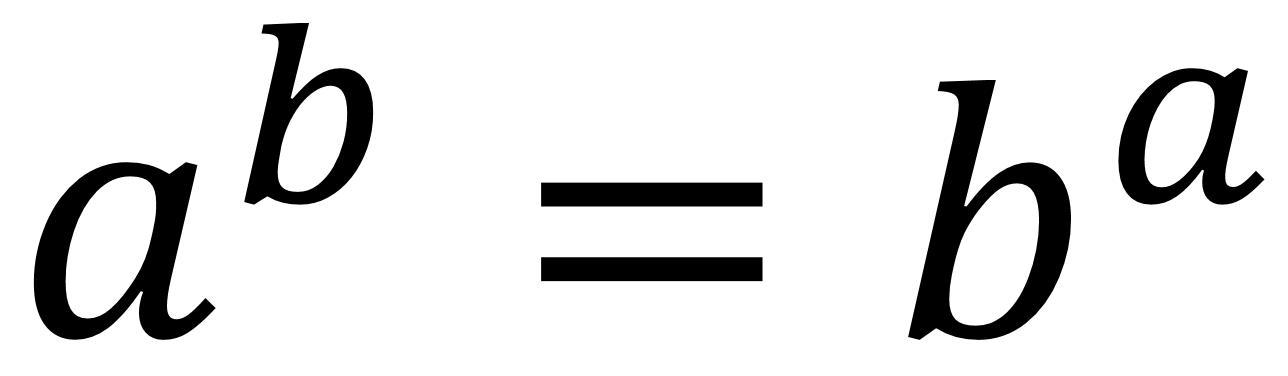# Equivalent Expressions with Powers

Directions: Find values for a and b that will make the expressions equivalent, assuming that a does not equal b.### Hint

How does each base’s factors affect the equivalence?

There are two possible answers. One is when a and b are 2 and 4, giving 2^4 = 4^2. The other was found by Shashi and is when a and b are -2 and -4, giving (-2)^(-4) = (-4)^(-2).

Source: Owen Kaplinsky

## Greatest Common Factor

Directions: Using the digits 0 to 9 at most one time each, place a digit …

1.2.Oh! I didn’t think of that!

3.•Robert Kaplinsky

Unfortunately, this won’t work because it says that a cannot equal b.

•Thanks for telling me this!

4.5.6.